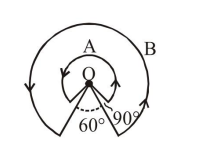# Solve this following

Question:

A wire A, bent in the shape of an arc of a circle, carrying a current of $2 \mathrm{~A}$ and having radius $2 \mathrm{~cm}$ and another wire B, also bent in the shape of arc of a circle, carrying a current of $3 \mathrm{~A}$ and having radius of $4 \mathrm{~cm}$, are placed as shown in the figure. The ratio of the magnetic fields due to the wires $\mathrm{A}$ and $\mathrm{B}$ at the common centre $\mathrm{O}$ is :1. $4: 6$

2. $6: 4$

3. $6: 5$

4. $2: 5$

Correct Option: , 3

Solution:

Given $\mathrm{i}_{\mathrm{A}}=2, \mathrm{r}_{\mathrm{A}}=2 \mathrm{~cm}, \theta_{\mathrm{A}}=2 \pi-\frac{\pi}{2}=\frac{3 \pi}{2}$

$\mathrm{i}_{\mathrm{B}}=3, \mathrm{r}_{\mathrm{B}}=4 \mathrm{~cm}, \theta_{\mathrm{B}}=2 \pi-\frac{\pi}{3}=\frac{5 \pi}{3}$

$\mathrm{B}=\frac{\mu_{0} \mathrm{I} \theta}{4 \pi \mathrm{R}}$

$\frac{B_{A}}{B_{B}}=\frac{I_{A}}{I_{B}} \times \frac{\theta_{A} R_{B}}{\theta_{B} R_{A}}=\frac{6}{5}$